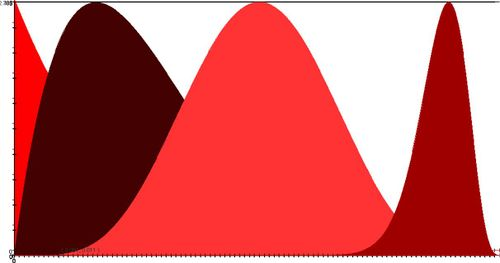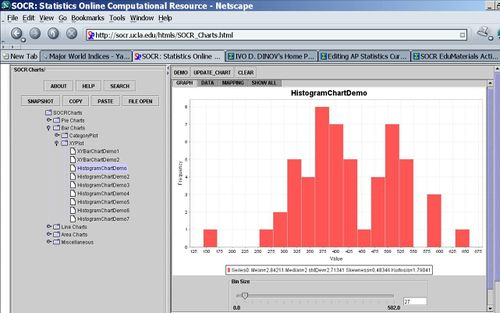# AP Statistics Curriculum 2007 EDA Shape

## General Advance-Placement (AP) Statistics Curriculum - Measures of Shape

### Definitions

• A distribution is unimodal if it has one mode. Unimodal distributions include:
• Bell shaped distributions (symmetric, Normal)
• Skewed right or skewed left
• We can use the mean and median to help interpret the shape of a distribution. For an unimodal distribution we have these properties:
• If mean = median, then the distribution is symmetric
• If mean > median, then the distribution is right skewed
• If mean < median, then the distribution is left skewed
• Note that depending on the protocol for computing the median, these rules may be violated for certain distributions. Some examples of such violations are included in this JSE paper.
• Multimodal distributions have two or more modes. Examples of multimodal distributions are:### Examples

What seems like a logical choice for the shape of the hot dog calorie data? Try looking at the histogram of the calories for the Hot-dogs dataset.### Activities

Collect data, draw the sample histogram or dot-plot and classify the shape of the distribution accordingly. Also, if unimodal, classify symmetry (symmetric, skewed right or skewed left).

• Data collected on height of randomly sampled college students.
• Data collected on height of randomly sampled female college students.
• The salaries of all persons employed by a large university.
• The amount of time spent by students on a difficult exam.
• The grade distribution on a difficult exam.

"-----